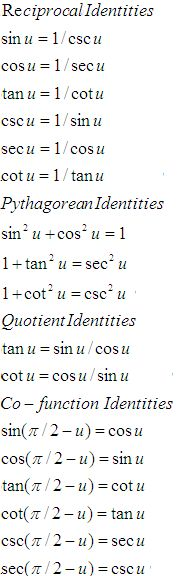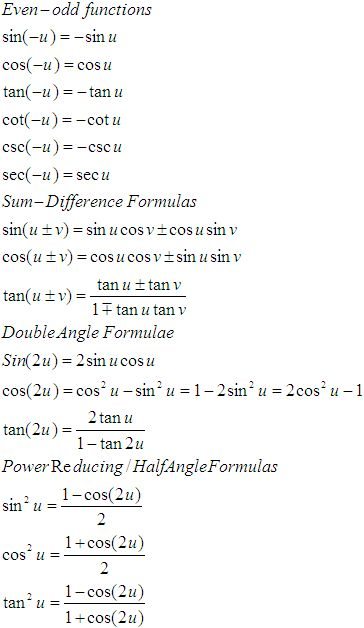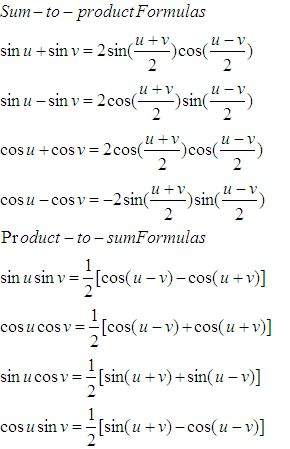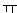Name: ___________________Date:___________________

 Email us to get an instant 20% discount on highly effective K-12 Math & English kwizNET Programs!

### High School Mathematics - 210.18 Trignometry Review

 Function 0o 30° 45° 60° 90° sin Ö0/2 Ö1/2 Ö2/2 Ö3/2 Ö4/2 cos Ö4/2 Ö3/2 Ö2/2 Ö1/2 0 tan 0 Ö3/3 1 Ö3 undefined sec 1 2Ö3/3 Ö2 2 undefined csc undefined 2 Ö2 2Ö3/3 1 cot undefined Ö3 1 Ö3/3 0

 sin cos tan 0 0 1 0 90 1 0 infinity 180 0 -1 0 270 -1 0 infinityFunctions of angles in all quadrants in terms of those in quadrant 1
 -A 900 ± A P/2 ± A 1800 ± A P ± A 2700 ± A 3P/2 ± A 3600 ± A 2P ± A sin -sinA cos A ±sin A -cosA ±sin A cos cos A ±sin A -cos A ±sin A cos A tan -tan A ±cot A ± tan A ±cot A ±tan A csc -csc A sec A ± csc A -sec A ±csc A sec sec A ± csc A -sec A ±csc A sec A cot -cot A ± tan A ± cot A ±tan A ± cot A

 Q 1: In triangle ABC, if b = 6, c = 4, cos A = 1/12, find a431/24.31/2 Q 2: In triangle ABC , if 2 cos A/a + cos B/b + 2 cos C/c = a/bc + b/ca. find angle A30 degrees45 degrees90 degrees Q 3: From the light house the angles of depression of two ships on opposite sides of the light house are observed to be 30o and 45o. If the height of the light house is 300 metres, find the distance between the ships if the line joining them passes through foot of the light house.809 metres800 metres819.6 metres Q 4: If in two circlesarcs of the same length subtend angles 60o and 75o at the centre, find the ratio of their radii.1:22:35:4 Q 5: What is the angle of elevation of the sun when the length of the shadow of a pole is 31/2 times the height of the pole?30o90o60o Q 6: Solve the triangle ABC given that A = 67o, b = 3 cms, c = 2 cms.a = 2 cm, B = 73oC = 39o 41la = 5 cm, B = 70o19l, C = 39o 41la = 2.88 cm, B = 73o19l, C = 39o 41l Q 7: Find the length of an arc of a circle of radius 10 cm which subtends an angle of 45o at the centre.5/47/43/4 Q 8: Find the radian measure of 15o./12/4/6 Question 9: This question is available to subscribers only! Question 10: This question is available to subscribers only!